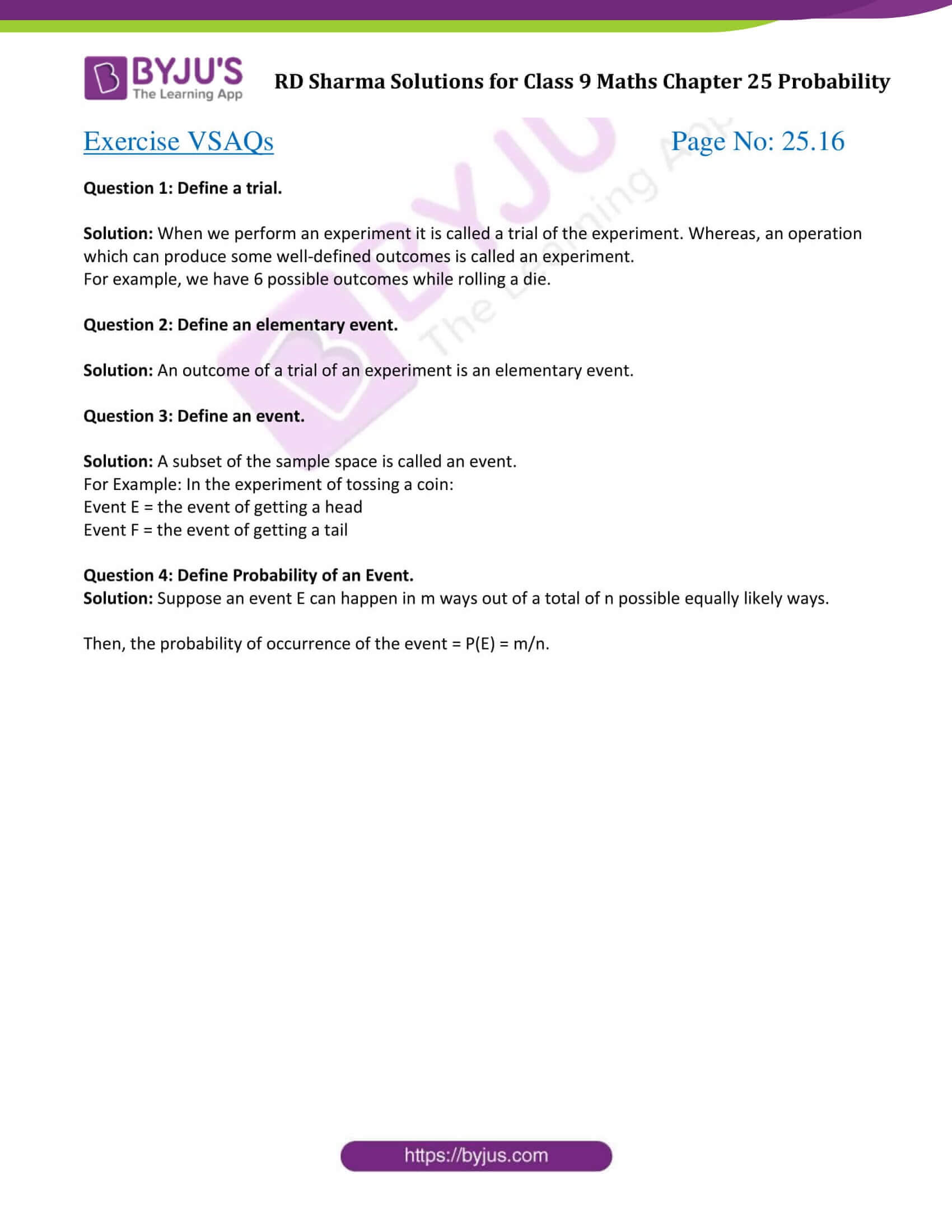# RD Sharma Solutions for Class 9 Maths Chapter 25 Probability Exercise VSAQs

Class 9 Chapter 25 – Probability Exercise VSAQs solutions are provided here. RD Sharma Solutions are outlined by subject matter experts. These exercise solutions involve step-by-step solutions to all questions in Class 9 RD Sharma textbook. This study material is helpful for the students while completing their homework or while preparing for their exams.

## Download PDF of RD Sharma Solutions for Class 9 Maths Chapter 25 Probability Exercise VSAQs### Access Answers to Maths RD Sharma Solutions for Class 9 Chapter 25 Probability Exercise VSAQs Page number 25.16

Exercise VSAQs Page No: 25.16

Question 1: Define a trial.

Solution: When we perform an experiment it is called a trial of the experiment. Whereas, an operation which can produce some well-defined outcomes is called an experiment.

For example, we have 6 possible outcomes while rolling a die.

Question 2: Define an elementary event.

Solution: An outcome of a trial of an experiment is an elementary event.

Question 3: Define an event.

Solution: A subset of the sample space is called an event.

For Example: In the experiment of tossing a coin:

Event E = the event of getting a head

Event F = the event of getting a tail

Question 4: Define Probability of an Event.

Solution: Suppose an event E can happen in m ways out of a total of n possible equally likely ways.

Then, the probability of occurrence of the event = P(E) = m/n.

## RD Sharma Solutions for Class 9 Maths Chapter 25 Probability Exercise VSAQs

RD Sharma Solutions Class 9 Maths Chapter 25 Probability Exercise VSAQs is based on following topics:

• Occurence of an event
• Empirical probability